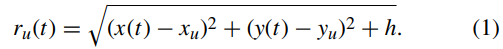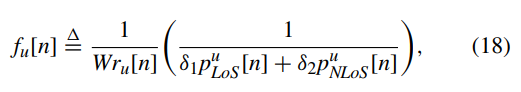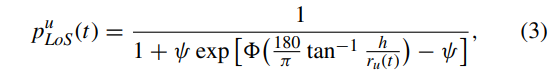# Error using cvx/sqrt (line 61) Disciplined convex programming error: Illegal operation: sqrt( {convex} )

Hi
I try to write this formula on matlab with cvx which give error that I wrote as topic
The formula is:I wrote code as this:

``````    for u = 1:U

for n = 1:N

ru = sqrt((x(n)-xu(u,:))^2+(y(n)-yu(u,:))^2+H))

end

end
``````

where xu and yu are 6by20 constant vector and x and y are1by20 variable vectors and H is height parameter which is constraint
this formula is the distance between 6 user and 1 UAV but i got that error how can I solve this error?
i try sth like:

``````ru = norm([X(1,n); Y(1,n); H] - [Xu(u,n); Yu(u,n); 0])
``````

is it ok? as i wrote like this I cant calculate inverse of ru.

Welcome to the forum!!

The formula using `norm` should work, but it should have `sqrt(H)` in place of `H` in the argument of `norm`.

By `inverse of ru`, I presume you mean`reciprocal`. That is not allowed in CVX, because `norm` is convex, not concave (as would be needed for `inv_pos` or `pow_p`) .So that raises the possibility that your optimization problem is not convex. I don’t know whether it is, because I haven’t seen what you intend to to with the reciprocal of `norm`, If the desired use is of the form `{affine expression}/norm(...) >= constant`, it could be reformulated as `{affine expression} >= constant*norm(...)`, which is a convex constraint and would be accepted by CVX.

So your first step is to prove your optimization problem is convex.

for clarification I send formulas and problem to see where this reciprocal used in formulation
first of all the problem, formulas and constraints are :

as seen ru used in eq. (3 and 18) directly which need reciprocal of ru.
bu[n] is binary decision variable and some parameters like( Phi,Psi,sigma_2, delta 1, delta 2) are constant and known also W in eq.18 is c/4pifc which are all known.

I really Thank you for answering. This problem take 4 months of my time.

also this is the article link : (IEEE Xplore Full-Text PDF:)

Where is there a reciprocal of norm?

In 18 ru used, As i wrote as this formulai got error so i wrote in norm format which i searched in internet.as be seen in 3 atan(h/ru) and in 18 1/w*ru

actually for writing formula of ru as be seen above in eq.1 i got error using sqrt so I wrote norm and again at this time i got error for calculation of h/ru to use in tangent inverse where is shown above in eq.3The carefully reread the paper, paying attention to what is the actual convex optimization problem being solved by CVX, and understanding what the optimization variables in that problem, and what the input data is, and where it comes from (including initial value, and updates based on previous CVX problem.

The paper uses SCA, which I am not a fan of, so don’t be surprised if even if you"correctly" implement the overall algorithm as described in the paper, the method might not always converge to anything, let alone an even local optimum of the original problem.

Thank you so much Mark for your help.

Have you handle this problem? I meet the same question to express the following equation.I am presuming h is noneagtive input data.
`norm([x(t)-xu;y(t)-yu;sqrt(h)])`

1 Like